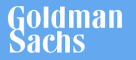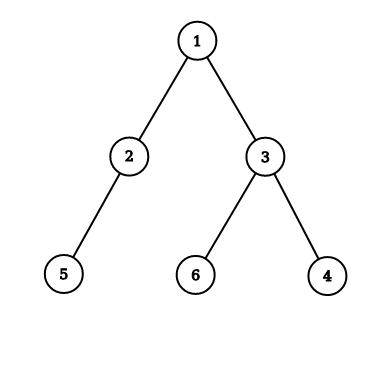New update is available. Click here to update.
Last Updated: 5 Dec, 2020

# Level Order Traversal

Easy## Problem statement#### Return the level-order traversal of the Binary Tree.

##### Example:
``````Input: Consider the following Binary Tree:
````````````Output:
Following is the level-order traversal of the given Binary Tree: [1, 2, 3, 5, 6, 4]
``````

##### Input Format:
``````The only line contains elements in the level order form. The line consists of values of nodes separated by a single space. In case a node is null, we take -1 in its place.

For example, the input for the tree depicted in the below image will be:
````````````1
2 3
4 -1 5 6
-1 7 -1 -1 -1 -1
-1 -1

Explanation :
Level 1 :
The root node of the tree is 1

Level 2 :
Left child of 1 = 2
Right child of 1 = 3

Level 3 :
Left child of 2 = 4
Right child of 2 = null (-1)
Left child of 3 = 5
Right child of 3 = 6

Level 4 :
Left child of 4 = null (-1)
Right child of 4 = 7
Left child of 5 = null (-1)
Right child of 5 = null (-1)
Left child of 6 = null (-1)
Right child of 6 = null (-1)

Level 5 :
Left child of 7 = null (-1)
Right child of 7 = null (-1)

The first not-null node(of the previous level) is treated as the parent of the first two nodes of the current level. The second not-null node (of the previous level) is treated as the parent node for the next two nodes of the current level and so on.

The input ends when all nodes at the last level are null(-1).

The sequence will be put together in a single line separated by a single space. Hence, for the above-depicted tree, the input will be given as:

1 2 3 4 -1 5 6 -1 7 -1 -1 -1 -1 -1 -1
``````

##### Output Format:
``````Return an array representing the level-order traversal of the given binary tree.
``````

##### Note :
``````You do not need to print anything; it has already been taken care of. Just implement the given function.
``````## Approaches

### 01 Approach#### Approach:

While traversing the binary tree in a level order way, we traverse the tree level by level, from left to right. This is essentially a breadth-first traversal of the binary tree. We will use a queue to maintain the order of the nodes that we need to traverse. Initially the queue will contain only the root of the tree. Then for each node, we will do the following:

• Pop the node in the front of the queue.
• Add its left child to the end of the queue.
• Add its right child to the end of the queue.
• Append the value of the current node to the path.

#### function levelOrder(TreeNode<int>* root)

1. Initialise empty array path
2. Initialise empty queue ‘q’
3. q.push(root)
4. while(!q.empty())
1. cur = q.pop()
2. Push value of current node to the path
3. if(cur->left)
1. q.push(cur->left)
4. if(cur->right)
1. q.push(cur->right)
5. return path